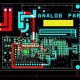# Best Tutorial for Electronics Components

In this tutorial, we are going to learn about electronics components

Electronics Components:

• These basic components use for development the electronics circuit.
• Without these components, circuit designs are never complete these are the main components are like – Resistors, diodes, capacitors, Integrated circuit etc.

For Electronics Circuit need the following are must requirement.

• First need the basic components such as capacitors, resistors, diode, transistor s etc.
• Power source: Mostly use the signal generator and DC power supplies.
• Measurement and analysis instruments: cathode ray Oscillations.
• Measurement and analysis instruments such as s cathode ray oscilloscope (CRO), millimeter etc.

Types of Components:

• Passive components
• Active Components

Passive Components – Passive components can store or maintains energy either in the form of current or voltage.

Below are the main passive components:

1. Resistor – A resistor is a two terminals passive electronic components which is use to oppose or limit the current into the circuit.

• By resistor we can reduce the current, divide the voltage, setup biasing of transistors etc.
• Ohm’s Law – If voltage applied across the terminal of resistor is directly proportional to the current flowing through

V = IR

Unit of resistance is OHM.

• Capacitor
• A device which is use to store energy in the form of electric field. Generally capacitors consist of two conducting plates that are separated by a dielectric material.
• If Q is the charge of conductor plates and V is the voltage between them, then the capacitance C of the capacitor is C = Q/V
• A Capacitor is generally used to block DC current and allow AC current. The other applications of capacitors are filters, timing circuits, power supplies and energy storing elements.
• There are many types of capacitors like polarized, non – polarized, ceramic, film electrolyte, poly etc.
• Inductor –
• It is device which store energy in the form of magnetic field. Inductor is nothing but a wire that is wound in form of a coil.
• Inductor is also a passive components and widely used in AC equipments like filters, chokes, tuned circuits etc.
• The core around which the coil is wound like air, iron, ferrite etc. It is determined the strength of the magnetic field. Inductor oppose the change in electric current through them and the changes in current will result in induction of voltage. When inductor discharge it change its polarity.